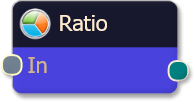#### Risk Disclosure

Futures, foreign currency and options trading contains substantial risk and is not for every investor. An investor could potentially lose all or more than the initial investment. Risk capital is money that can be lost without jeopardizing ones financial security or lifestyle. Only risk capital should be used for trading and only those with sufficient risk capital should consider trading. Past performance is not necessarily indicative of future results. View full risk disclosure.

## Ratio NodeThe Ratio logic node takes each of the inputs and calculates a weighted average of the values to produce an output. This is useful for implementing a system where the optimal combination of influence for each criteria is unknown. You can use the Ratio logic node in conjunction with theoutput multiplier (found on the weight slider) to fine tune the relative effect each criteria will have on the final output.

Ratio logic nodes take full advantage of the fuzzy output generated by the solvers. In a fuzzy logic system where values are presented in a range between 0 and 1, the Ratio logic node allows you to combine the influence of your criteria in an analog fashion. This is best used for those systems where you have outlined a number of positive factors contributing to a trade signal, but there are instances where a deficiency in one criteria can be compensated by another. The Ratio logic node emulates the discretionary trader who examines a variety of positive (or negative) factors weighing the contribution of each of them before executing a trade.

## Example

Suppose you had several criteria but needed the flexibility to change how much each individual criteria contributes to the final output.

• Criteria #1
• Criteria #2
• Criteria #3
• Criteria #4

After adding the solvers to the Logic board workspace, add a Ratio node. Then connect the outputs of each solver node to the input points on the Ratio node, and finally connect the Ratio node itself to the Result node. You should notice immediately that BloodHound’s output appears to be a blend or average value of all the criteria combined.

At this point you can manipulate the output multiplier for each solver node to increase or reduce the influence of each solver separately. Suppose that you would like to increase the influence of criteria 1 so that it’s favorable value was twice as meaningful as the other criteria. To do this, you would click on the Ratio node to select it. On the right hand pane, you will see a list of weight sliders, each pertaining to the solver(along with the weight slider for the Ratio node itself at the top). Find the weight slider for criteria 1, and set the output multiplier to 2.0. Criteria 1 is now weighted as twice as significant as the other criteria, giving it more influence over the Ratio node’s final output.

As you change the influence in real-time mode, you will notice that BloodHound immediately updates the chart with the new weightings. This allows you to make tweaks  and visually inspect the results until you are satisfied. Another tip to observe is that you can assign a plot color to each of the solvers to inspect their raw values while you are manipulating their weights. See the section on weight slider control to learn how to do this.

After changing the weighting for your solvers, suppose that you now have the following output multiplier(weights) values for each input to the Ratio node:

Criteria Weight
Input #1 1.5
Input #2 2
Input #3 0.5
Input #4 1

The resulting calculation on each bar would be derived from the following formula (which is simply a weighted average calculation, using the Output Multiplier as the weighting):## Example Video

This video is a snippet from the workshop on January 6, 2017. Find all workshop recordings here.